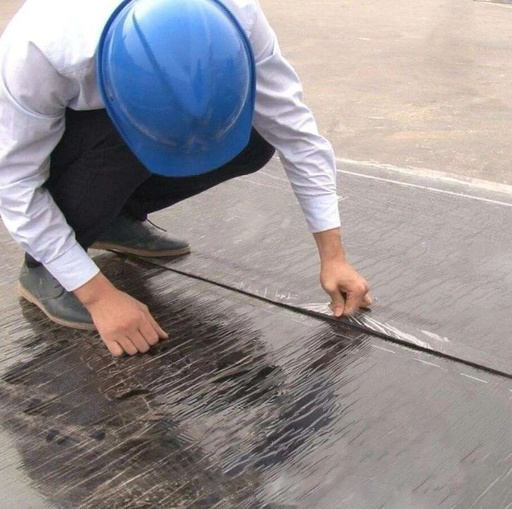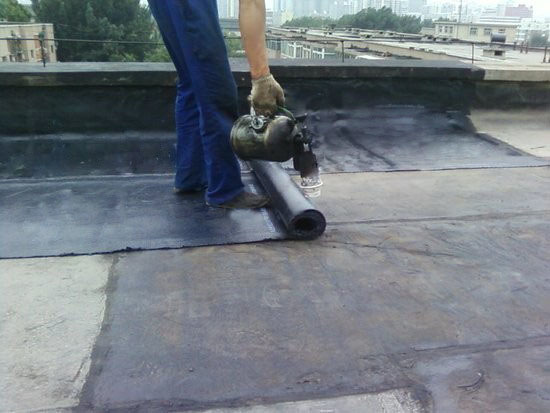|

# 防水卷材施工全流程:从材料进场检验到保护层施工

1、对材料的外观、品种、规格、包装、尺寸和数量等进行检查，并经监理单位或建设单位代表检查确认，形成相应验收记录。

2、材料进场必须同时提供对应的出厂合格证、材料出厂检测报告等证明资料，并经监理单位或建设单位代表检查确认。

3、材料进场后应执行见证取样送检制度，检验项目全部指标达到标准规定时，即为合格；若有一项指标不符合标准规定，应在受检产品中重新取样进行该项指标复验，复验结果符合标准规定，则判定改批材料为合格：（1）抽样数量：大于1000卷抽5卷，每500～1000卷抽4卷，100～499卷抽3卷，100卷以下抽2卷，进行规格尺寸和外观质量质检。在外观质量检验合格的卷材中，任取一卷作物理性能检验。

（2）地下工程卷材外观质量检验：断裂、折皱、孔洞、剥离、边缘不整齐，胎体露白、未浸透，撒布材料粒度、颜色，每卷卷材的接头。

（3）地下工程卷材物理性能检验：可溶物含量，拉力，延伸率，低温柔度，热老化后低温柔度，不透水性。

1、贮存时应避免日晒雨淋，注意通风。

2、不同材料分类码放。

3、在正常贮存条件下，保质期12个月。

4、贮存温度不应高于50℃，立放贮存只能单层。1、防水基层应坚实、平整、干净、干燥、无浮浆、无起砂、裂缝等现象，表面的灰尘、油污、颗粒等杂物应清理干净并会同监理工程师严格执行交接检查。

2、平立面交接处、转折处、阴阳角、管根等均应做成均匀一致、平整光滑的圆角，圆弧半径不小于50mm。

3、与基层连接的管件、支架等必须安装牢固，在防水层施工前先将预留管道安装牢固，且必须安装完毕方可进行防水层的施工。

4、所有管道、支架等表面要进行增糙或防锈处理。

5、排水坡度应满足要求。1、基层清理：防水层的基层应满足防水层的施工要求后再移交；

2、涂刷基层处理剂：要涂刷均匀，不得漏刷或露底。干燥后方可施行附加卷材施工。涂刷基层处理剂后的基层应尽快铺贴卷材，以免受到二次灰尘污染；

3、细部附加增强层处理：在两面转角、三面阴阳角等部位进行附加增强处理，附加层要求无空鼓，并压实铺牢。附加层卷材为500mm宽，一般部位附加层应满粘结在基层上；

4、弹线：在已处理好并干燥的基层表面，按照所选卷材的宽度，留出搭接缝尺寸（长短边均为100mm），将铺贴卷材的基准线弹好；按此基准线进行卷材预铺，释放应力，然后将卷材重新打卷进行卷材铺贴施工；

5、大面铺贴卷材：将起始端卷材粘牢后，持火焰喷灯对着待铺的整卷卷材，使喷灯距卷材及基层加热处0.3～0.5m施行往复移动烘烤，应加热均匀，及时推滚卷材进行粘铺，后随一人施行排气压实工序。铺贴后卷材应平整、顺直，搭接尺寸正确，不得扭曲。

6、接缝处理：用喷灯充分烘烤搭接边上层卷材底面和下层卷材上表面沥青涂盖层，必须保证搭接处卷材间的沥青密实熔合，且有熔融沥青从边端溢出，形成匀质沥青条。1、地下工程防水层验收：

（1）卷材防水层在转角处、变形缝、施工缝、穿墙管等部位做法必须符合设计要求。

（2）卷材防水层的搭接缝应粘贴或焊接牢固，密封应严密，不得有扭曲、褶皱、翘边和起泡等缺陷。（卷材防水层及搭接缝部位如出现上述问题，需将问题部位裁剪清理干净后重新修补，修补时新做防水卷材应与原防水卷材形成一个整体并保证有效搭接尺寸，搭接应≥150mm。）

（3）卷材搭接宽度的允许偏差应为-10mm。

2、屋面工程防水层验收：

（1）卷材防水层不得有渗漏和积水现象。

（2）卷材防水层在檐口、檐沟、天沟、水落口、泛水、变形缝和伸出屋面管道的防水构造，应符合设计要求。

（3）卷材防水层的搭接缝应粘贴或焊接牢固，密封应严密，不得有扭曲、褶皱和翘边。（卷材防水层及搭接缝部位如出现上述问题，需将问题部位裁剪清理干净后重新修补，修补时新做防水卷材应与原防水卷材形成一个整体并保证有效搭接尺寸，搭接应≥150mm。）

（4）卷材防水层的收头应与基层粘结，钉压应牢固，密封应严密。

（5）卷材防水层的铺贴方向应正确，卷材搭接宽度的允许偏差应为-10mm。

`声明：本文由入驻焦点开放平台的作者撰写，除焦点官方账号外，观点仅代表作者本人，不代表焦点立场错误信息举报电话： 400-099-0099，邮箱：jubao@vip.sohu.com，或点此进行意见反馈，或点此进行举报投诉。`A B C D E F G H J K L M N P Q R S T W X Y Z
A - B - C - D - E
• A
• 鞍山
• 安庆
• 安阳
• 安顺
• 安康
• 澳门
• B
• 北京
• 保定
• 包头
• 巴彦淖尔
• 本溪
• 蚌埠
• 亳州
• 滨州
• 北海
• 百色
• 巴中
• 毕节
• 保山
• 宝鸡
• 白银
• 巴州
• C
• 承德
• 沧州
• 长治
• 赤峰
• 朝阳
• 长春
• 常州
• 滁州
• 池州
• 长沙
• 常德
• 郴州
• 潮州
• 崇左
• 重庆
• 成都
• 楚雄
• 昌都
• 慈溪
• 常熟
• D
• 大同
• 大连
• 丹东
• 大庆
• 东营
• 德州
• 东莞
• 德阳
• 达州
• 大理
• 德宏
• 定西
• 儋州
• 东平
• E
• 鄂尔多斯
• 鄂州
• 恩施
F - G - H - I - J
• F
• 抚顺
• 阜新
• 阜阳
• 福州
• 抚州
• 佛山
• 防城港
• G
• 赣州
• 广州
• 桂林
• 贵港
• 广元
• 广安
• 贵阳
• 固原
• H
• 邯郸
• 衡水
• 呼和浩特
• 呼伦贝尔
• 葫芦岛
• 哈尔滨
• 黑河
• 淮安
• 杭州
• 湖州
• 合肥
• 淮南
• 淮北
• 黄山
• 菏泽
• 鹤壁
• 黄石
• 黄冈
• 衡阳
• 怀化
• 惠州
• 河源
• 贺州
• 河池
• 海口
• 红河
• 汉中
• 海东
• 怀来
• I
• J
• 晋中
• 锦州
• 吉林
• 鸡西
• 佳木斯
• 嘉兴
• 金华
• 景德镇
• 九江
• 吉安
• 济南
• 济宁
• 焦作
• 荆门
• 荆州
• 江门
• 揭阳
• 金昌
• 酒泉
• 嘉峪关
K - L - M - N - P
• K
• 开封
• 昆明
• 昆山
• L
• 廊坊
• 临汾
• 辽阳
• 连云港
• 丽水
• 六安
• 龙岩
• 莱芜
• 临沂
• 聊城
• 洛阳
• 漯河
• 娄底
• 柳州
• 来宾
• 泸州
• 乐山
• 六盘水
• 丽江
• 临沧
• 拉萨
• 林芝
• 兰州
• 陇南
• M
• 牡丹江
• 马鞍山
• 茂名
• 梅州
• 绵阳
• 眉山
• N
• 南京
• 南通
• 宁波
• 南平
• 宁德
• 南昌
• 南阳
• 南宁
• 内江
• 南充
• P
• 盘锦
• 莆田
• 平顶山
• 濮阳
• 攀枝花
• 普洱
• 平凉
Q - R - S - T - W
• Q
• 秦皇岛
• 齐齐哈尔
• 衢州
• 泉州
• 青岛
• 清远
• 钦州
• 黔南
• 曲靖
• 庆阳
• R
• 日照
• 日喀则
• S
• 石家庄
• 沈阳
• 双鸭山
• 绥化
• 上海
• 苏州
• 宿迁
• 绍兴
• 宿州
• 三明
• 上饶
• 三门峡
• 商丘
• 十堰
• 随州
• 邵阳
• 韶关
• 深圳
• 汕头
• 汕尾
• 三亚
• 三沙
• 遂宁
• 山南
• 商洛
• 石嘴山
• T
• 天津
• 唐山
• 太原
• 通辽
• 铁岭
• 泰州
• 台州
• 铜陵
• 泰安
• 铜仁
• 铜川
• 天水
• 天门
• W
• 乌海
• 乌兰察布
• 无锡
• 温州
• 芜湖
• 潍坊
• 威海
• 武汉
• 梧州
• 渭南
• 武威
• 吴忠
• 乌鲁木齐
X - Y - Z
• X
• 邢台
• 徐州
• 宣城
• 厦门
• 新乡
• 许昌
• 信阳
• 襄阳
• 孝感
• 咸宁
• 湘潭
• 湘西
• 西双版纳
• 西安
• 咸阳
• 西宁
• 仙桃
• 西昌
• Y
• 运城
• 营口
• 盐城
• 扬州
• 鹰潭
• 宜春
• 烟台
• 宜昌
• 岳阳
• 益阳
• 永州
• 阳江
• 云浮
• 玉林
• 宜宾
• 雅安
• 玉溪
• 延安
• 榆林
• 银川
• Z
• 张家口
• 镇江
• 舟山
• 漳州
• 淄博
• 枣庄
• 郑州
• 周口
• 驻马店
• 株洲
• 张家界
• 珠海
• 湛江
• 肇庆
• 中山
• 自贡
• 资阳
• 遵义
• 昭通
• 张掖
• 中卫

1室1厅1厨1卫1阳台

1
2
3
4
5

0
1
2

1

1

0
1
2
3报名成功，资料已提交审核A B C D E F G H J K L M N P Q R S T W X Y Z
A - B - C - D - E
• A
• 鞍山
• 安庆
• 安阳
• 安顺
• 安康
• 澳门
• B
• 北京
• 保定
• 包头
• 巴彦淖尔
• 本溪
• 蚌埠
• 亳州
• 滨州
• 北海
• 百色
• 巴中
• 毕节
• 保山
• 宝鸡
• 白银
• 巴州
• C
• 承德
• 沧州
• 长治
• 赤峰
• 朝阳
• 长春
• 常州
• 滁州
• 池州
• 长沙
• 常德
• 郴州
• 潮州
• 崇左
• 重庆
• 成都
• 楚雄
• 昌都
• 慈溪
• 常熟
• D
• 大同
• 大连
• 丹东
• 大庆
• 东营
• 德州
• 东莞
• 德阳
• 达州
• 大理
• 德宏
• 定西
• 儋州
• 东平
• E
• 鄂尔多斯
• 鄂州
• 恩施
F - G - H - I - J
• F
• 抚顺
• 阜新
• 阜阳
• 福州
• 抚州
• 佛山
• 防城港
• G
• 赣州
• 广州
• 桂林
• 贵港
• 广元
• 广安
• 贵阳
• 固原
• H
• 邯郸
• 衡水
• 呼和浩特
• 呼伦贝尔
• 葫芦岛
• 哈尔滨
• 黑河
• 淮安
• 杭州
• 湖州
• 合肥
• 淮南
• 淮北
• 黄山
• 菏泽
• 鹤壁
• 黄石
• 黄冈
• 衡阳
• 怀化
• 惠州
• 河源
• 贺州
• 河池
• 海口
• 红河
• 汉中
• 海东
• 怀来
• I
• J
• 晋中
• 锦州
• 吉林
• 鸡西
• 佳木斯
• 嘉兴
• 金华
• 景德镇
• 九江
• 吉安
• 济南
• 济宁
• 焦作
• 荆门
• 荆州
• 江门
• 揭阳
• 金昌
• 酒泉
• 嘉峪关
K - L - M - N - P
• K
• 开封
• 昆明
• 昆山
• L
• 廊坊
• 临汾
• 辽阳
• 连云港
• 丽水
• 六安
• 龙岩
• 莱芜
• 临沂
• 聊城
• 洛阳
• 漯河
• 娄底
• 柳州
• 来宾
• 泸州
• 乐山
• 六盘水
• 丽江
• 临沧
• 拉萨
• 林芝
• 兰州
• 陇南
• M
• 牡丹江
• 马鞍山
• 茂名
• 梅州
• 绵阳
• 眉山
• N
• 南京
• 南通
• 宁波
• 南平
• 宁德
• 南昌
• 南阳
• 南宁
• 内江
• 南充
• P
• 盘锦
• 莆田
• 平顶山
• 濮阳
• 攀枝花
• 普洱
• 平凉
Q - R - S - T - W
• Q
• 秦皇岛
• 齐齐哈尔
• 衢州
• 泉州
• 青岛
• 清远
• 钦州
• 黔南
• 曲靖
• 庆阳
• R
• 日照
• 日喀则
• S
• 石家庄
• 沈阳
• 双鸭山
• 绥化
• 上海
• 苏州
• 宿迁
• 绍兴
• 宿州
• 三明
• 上饶
• 三门峡
• 商丘
• 十堰
• 随州
• 邵阳
• 韶关
• 深圳
• 汕头
• 汕尾
• 三亚
• 三沙
• 遂宁
• 山南
• 商洛
• 石嘴山
• T
• 天津
• 唐山
• 太原
• 通辽
• 铁岭
• 泰州
• 台州
• 铜陵
• 泰安
• 铜仁
• 铜川
• 天水
• 天门
• W
• 乌海
• 乌兰察布
• 无锡
• 温州
• 芜湖
• 潍坊
• 威海
• 武汉
• 梧州
• 渭南
• 武威
• 吴忠
• 乌鲁木齐
X - Y - Z
• X
• 邢台
• 徐州
• 宣城
• 厦门
• 新乡
• 许昌
• 信阳
• 襄阳
• 孝感
• 咸宁
• 湘潭
• 湘西
• 西双版纳
• 西安
• 咸阳
• 西宁
• 仙桃
• 西昌
• Y
• 运城
• 营口
• 盐城
• 扬州
• 鹰潭
• 宜春
• 烟台
• 宜昌
• 岳阳
• 益阳
• 永州
• 阳江
• 云浮
• 玉林
• 宜宾
• 雅安
• 玉溪
• 延安
• 榆林
• 银川
• Z
• 张家口
• 镇江
• 舟山
• 漳州
• 淄博
• 枣庄
• 郑州
• 周口
• 驻马店
• 株洲
• 张家界
• 珠海
• 湛江
• 肇庆
• 中山
• 自贡
• 资阳
• 遵义
• 昭通
• 张掖
• 中卫• 手机• 分享
• 设计
免费设计
• 计算器
装修计算器
• 入驻
合作入驻
• 联系
联系我们
• 置顶
返回顶部